# Trigonometric Functions of Acute AnglesPage 1

#### WATCH ALL SLIDES

Slide 1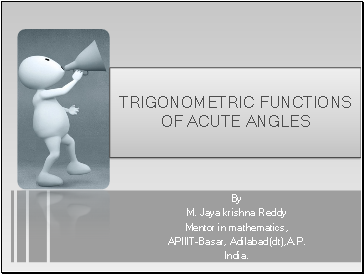## Trigonometric functions of acute angles

By

M. Jaya krishna Reddy

Mentor in mathematics,

India.

Slide 2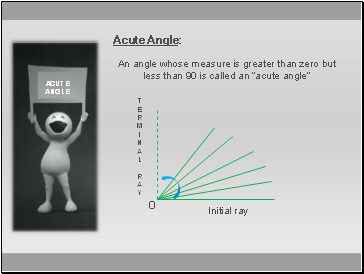## Acute Angle

An angle whose measure is greater than zero but less than 90 is called an “acute angle”

T

E

R

M

I

N

A

L

R

A

Y

Slide 3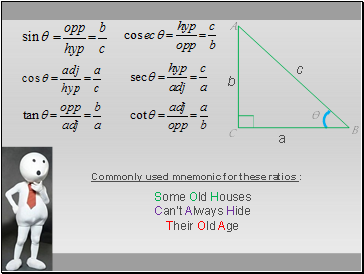Some Old Houses Can’t Always Hide

Their Old Age

Commonly used mnemonic for these ratios :

Ѳ

c

a

b

B

C

A

Slide 4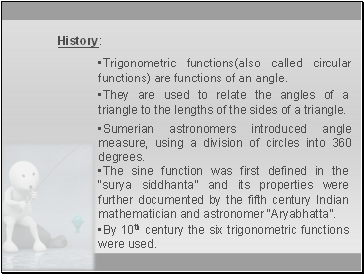## History

Trigonometric functions(also called circular functions) are functions of an angle.

:

They are used to relate the angles of a triangle to the lengths of the sides of a triangle.

Sumerian astronomers introduced angle measure, using a division of circles into 360 degrees.

The sine function was first defined in the “surya siddhanta” and its properties were further documented by the fifth century Indian mathematician and astronomer “Aryabhatta”.

By 10th century the six trigonometric functions were used.

Slide 5## Applications

In 240 B.C. a mathematician named “Eratosthenes” discovered the radius of the earth as 4212.48 miles using trigonometric functions

In 2001 a group of European astronomers did an experiment by using trigonometric functions and they got all the measurement, they calculate the Venus was about 105,000,000 km away from the sun and the earth was about 150, 000, 000 km away.

Optics and statics are 2 early fields of Physics that use trigonometry.

It is also the foundation of the practical art of surveying

Slide 61. Prove that

2. Prove that

Sol:

Sol:

Slide 7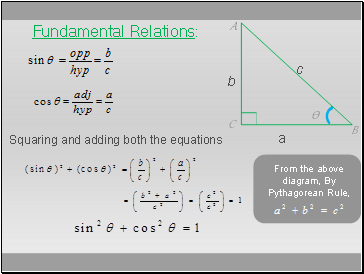## Fundamental Relations

Squaring and adding both the equations

Ѳ

c

a

b

C

A

B

Slide 8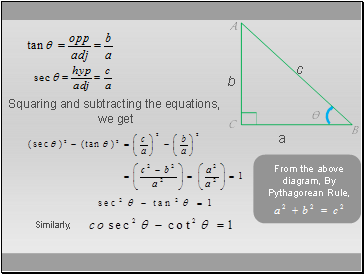Squaring and subtracting the equations,

we get

Similarly,

Ѳ

c

a

b

C

A

B

Slide 9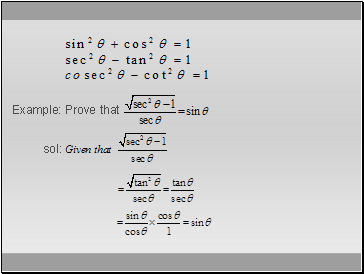Example: Prove that

sol:

Slide 10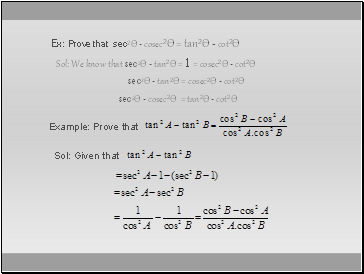Ex: Prove that sec2Ѳ - cosec2Ѳ = tan2Ѳ - cot2Ѳ

Sol: We know that sec2Ѳ - tan2Ѳ = 1 = cosec2Ѳ - cot2Ѳ

Go to page:
1  2